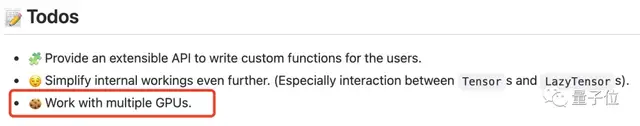# 1行代码消除PyTorch的CUDA内存溢出报错，这个GitHub项目刚发布就揽星600+

“大快人心”

CUDA error: out of memory.# 一行代码解决内存溢出错误

``````pip install koila
``````

``````# A batch of MNIST image
input = torch.randn(8, 28, 28)

# A batch of labels
label = torch.randn(0, 10, )

class NeuralNetwork(Module):
def __init__(self):
super(NeuralNetwork, self).__init__()
self.flatten = Flatten()
self.linear_relu_stack = Sequential(
Linear(28 * 28, 512),
ReLU(),
Linear(512, 512),
ReLU(),
Linear(512, 10),
)

def forward(self, x):
x = self.flatten(x)
logits = self.linear_relu_stack(x)
return logits``````

``````loss_fn = CrossEntropyLoss()

# Calculate losses
out = nn(t)
loss = loss_fn(out, label)

# Backward pass
loss.backward()
``````

koila就能自动帮你计算剩余的GPU内存并使用正确的batch size了。

``input = lazy(torch.randn(8, 28, 28), batch=0)``

# 灵感来自TensorFlow的静态/懒惰评估

“CUDA error: out of memory”这个报错通常发生在前向传递（forward pass）中，因为这时需要保存很多临时变量。

koila的灵感来自TensorFlow的静态/懒惰评估（static/lazy evaluation）。NO。

koila灵活又轻量，只需一行代码就能解决问题，非常“大快人心”有没有。ps. koila作者是一位叫做RenChu Wang的小哥。https://github.com/rentruewang/koila